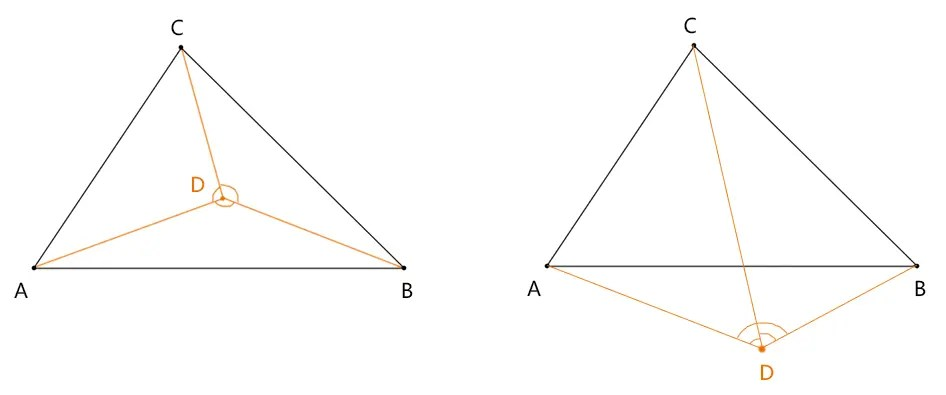# Graphic selection-grid selection

Posted May 26, 20203 min read

Mesh selection, as the name implies, is to select polygons after turning them into meshes(this method only applies to polygons, if they are curved, we have to segment them).In this way, the grid selection is divided into two steps:

1. Decompose the polygon into multiple triangles.
2. Determine whether the mouse point is in the triangle.

Let's start with the most basic judgment whether the mouse point is in the triangle. We can use the sum of the angle between the mouse point and the other vertices of the triangle to judge.• Point D is in ABC: ADB + BDC + CDA = 360 °
• Point D is not in ABC: ADB + BDC + CDA <360 °

Next, let s talk about how to calculate the angle based on three points, such as mon

First draw a corner based on three points:

``````const [m, o, n]= [
new Vector2(300,50),
new Vector2(300,200),
new Vector2(500,200),``````

];

``````const poly = new Poly({
stroke:true,
vertices:[m, o, n]
});
poly.draw(ctx);``````1. Zero the vertex o of mon:

m.x- = o.x;
m.y- = o.y;
n.x- = o.x;
n.y- = o.y;

1. According to the cosine theorem, the cosine value of mon can be calculated based on the dot product of point m times point n, the length of om times the length of on

const dot =(m.x * n.x + m.y * n.y);
const len = Math.sqrt(m.x * m.x + m.y * m.y) * Math.sqrt(n.x * n.x + n.y * n.y);
const cosTheta = dot/len;

1. Find mon according to the inverse cosine method acos(), which is the following theta

const theta = Math.acos(cosTheta);

Math.acos() can automatically limit the radians obtained from cosine to \ [0, Math.PI ].
If we use Math.atan2(y, x), we will get the radian based on the positive direction of the x-axis, and when the y value is negative, the value of atan2(y, x) is also a negative number, which is not suitable for the sum of angle .
As for the dot product formula involved here, this is pure mathematics, everyone first needs to know its usage, we have to start a chapter for it later:[Detailed explanation of dot product formula]1190000022748283)

After we know how to find an angle, we can then find the sum of the angles of ADB + BDC + CDA. If the sum is less than 360 °, it is outside the triangle, otherwise it is in the triangle.
I encapsulated this method in the Vector2d class:

``````inTriangle(p0, p1, p2) {
const [a0, a1, a2]= [
p0.includedAngleTo(this, p1),
p1.includedAngleTo(this, p2),
p2.includedAngleTo(this, p0),
];
const sum = a0 + a1 + a2;
return Math.PI * 2-sum <= 0.01;
}``````

Note:0.01 is a number used for tolerance. The computer's calculation of floating point numbers is not absolutely accurate, so I did not directly use Math.PI \ * 2 === sum to make the judgment, and let the mouse point be selected as long as it is close to a certain range of the triangle.

p1.includedAngleTo(p2, p3) is the way to find p1p2p3:

``````includedAngleTo(v1, v2) {
const [s1, s2]= [
this.clone(). sub(v1),
v2.clone(). sub(v1),
];
return s1.includedAngle(s2);
}``````

p1.includedAngle(p2) finds the angle after the vertex of the angle returns to zero

``````includedAngle(v) {
return Math.acos(this.clone(). dot(v)/(this.length() * v.length()));
}``````

dot() is the dot product method

``````dot(v) {
return this.x * v.x + this.y * v.y;
}``````

length() is the method to find the length of the vector

``````length() {
return Math.sqrt(this.x * this.x + this.y * this.y);
}``````

How to use inTriangle():

``````const poly = new Poly({
stroke:true,
close:true,
vertices:[
new Vector2(50,50),
new Vector2(450,50),
new Vector2(250,200),
]
});
poly.draw(ctx);

const p = new Vector2(150,100);
ctx.beginPath();
ctx.arc(p.x, p.y, 3,0, Math.PI * 2);
ctx.fill();
const bool = inTriangle(p, ... poly.vertices);
console.log(bool);``````

If the above bool is true, it means that the point is in the triangle.

We talked about the method of judging whether the point is in a triangle. Let s talk about the next chapter:[Graphic Selection-Polygon Meshing]